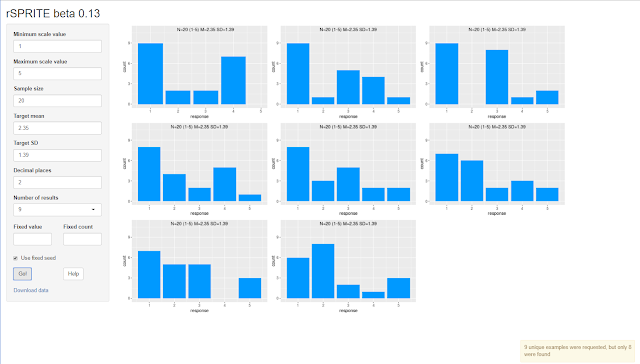## 31 May 2018

### How SPRITE works: a step-by-step introduction

Our preprint about SPRITE went live a few hours ago. I encourage you to read it, but not everyone will have the time, so here is a simple (I hope) explanation of what we're trying to do.

Before we start, I suggest that you open this Google spreadsheet and either make a copy or download an Excel version (both of these options are in the File menu) so you can follow along.

Imagine that you have read in an article that N=20 people responded to a 1–5 Likert-type item with a mean of 2.35 and an SD of 1.39. Here's how you could test whether that's possible:

1. Make a column of 20 random numbers in the range 1–5 and have your spreadsheet software display their mean and SD. Now we'll try and get the mean and SD to match the target values.

2. If the mean is less than the target mean (2.35), add 1 to one of the numbers that isn't a 5 (the maximum on the scale). If the mean is greater than the target mean, subtract 1 from one of the numbers that isn't a 1. Repeat this step until the mean matches the target mean.

3. If the SD doesn't match the target SD, select a pair of numbers from the list. Call the smaller number A and the larger one B (if they are identical, either can be A or B). If the SD is currently smaller than the target SD, subtract 1 from A and add 1 to B. If the SD is currently larger than the target SD, add 1 to A and subtract 1 from B. Repeat this step until the SD matches the target SD. (Not all pairs of numbers are good choices, as you will see if you play around a bit with the spreadsheet, but we can ignore that for the moment.)

Let's go through this in the spreadsheet; I hope you'll see that it's quite simple.

Here's the spreadsheet. Cells B2 and B3 contain the target mean and SD. Cells D2 and D3 contain the current mean and SD of the test data, which are the 20 numbers in cells D5 through D24. Cells C2 and C3 contain the difference between the current and target mean and SD, respectively. When that difference drops to 0.005 or less (which means that the numbers are equal, within the limits of rounding), these two cells will change colour. (For some reason, they turn green in Google Sheets but blue in my copy of Excel.)

In this spreadsheet, most of the work has already been done. The mean is 2.30 and the target is 2.35, so if you increase one value by 1 (say, D11, from 1 to 2), the mean will go to 2.35 and cell C1 will change colour. That's step 2 completed.

For the SD, observe that after you fixed the mean by changing D11, the SD became 1.31, which is smaller than the target. So you want to increase the SD, which means pushing two values further apart. For example, change D12 from 2 to 1 and D13 from 2 to 3. The mean will be unchanged, but now the SD is 1.35; changing two 2s to a 1 and a 3 increased the SD by 0.04, which is the amount that the SD is still short of the target. So let's do the same operation again. Change D14 from 2 to 1 and D15 from 2 to 3. You should now have an SD of 1.39, equal to the target value, and cell C2 should have changed colour. Step 3 is now completed.

Congratulations, you just found a SPRITE solution! That is, the list of values (after sorting)
1,1,1,1,1,1,1,1,2,2,2,3,3,3,3,3,4,4,5,5
has a mean of 2.35 and an SD of 1.39, and could have been the combination that gave the result that you were trying to reproduce from the article.

Not every swap of two values gives the same result, however. Let's back up a little by changing D15 from 3 back to 2 and D14 from 1 back to 2 (so the SD should now be back to 1.35). Now change cell D20 from 3 to 2 and cell D21 from 4 to 5. The mean is still OK, but the SD has overshot the target value of 1.39 and is now 1.42. So this means that
1,1,1,1,1,1,1,2,2,2,2,2,2,3,3,3,4,5,5,5
is not a valid solution.

There are eight unique solutions (I checked with CORVIDS); rSPRITE will usually find all eight, although it doesn't always get 100% of possible solutions in more complex cases. If playing with numbers like this is your idea of fun, you could try and find more solutions by hand. Here's the spoiler picture, with the solution we found earlier right in the middle:Basically, that's all there is to it. SPRITE is just software that does this adding and swapping, with a few extra subtleties, very fast. It's the computer version of some checks that James Heathers and I first started doing in late 2015 when we were looking at some dodgy-looking articles. But we certainly aren't the first people who have had this idea to see if means/SD combinations are possible; it really isn't rocket science.